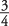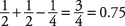## Double-Counting

By using the addition rule in a situation that is not mutually exclusive, you are double‐counting. One way of realizing that you are double‐counting is to use the classic theory of probability: List all the different outcomes when flipping a coin twice and assess the ratio of favorable outcomes to total outcomes (see Table 1).There are four total outcomes. Three of the outcomes have at least one head; therefore, the probability of throwing at least one head in two flips is, or 0.75, not 1. But if you had used the addition rule, you would have added the two heads from the first flip to the two heads from the second flip and gotten four heads in four flips,. But the two heads in that first pair constitute only one outcome; so, by counting both heads for that outcome, you are double‐counting because this is the joint‐occurrence outcome that is not mutually exclusive.

To use the addition rule in a non‐mutually‐exclusive situation, you must subtract any events that double‐count. In this case:The notation, therefore, for at least one favorable occurrence in two events is

P( AB) = P( A) + P( B) – P( AB)

which is read: The probability of at least one of the events A or B equals the probability of A plus the probability of B minus the probability of their joint occurrence. (Note that if they are mutually exclusive, then P( AB)—the joint occurrence—equals 0, and you simply add the two probabilities.)

##### Example 1
What is the probability of drawing either a spade or an ace from a deck of cards?

The probability of drawing a spade is; the probability of drawing an ace is. But the probability of their joint occurrence (an ace of spades) is. Thus,